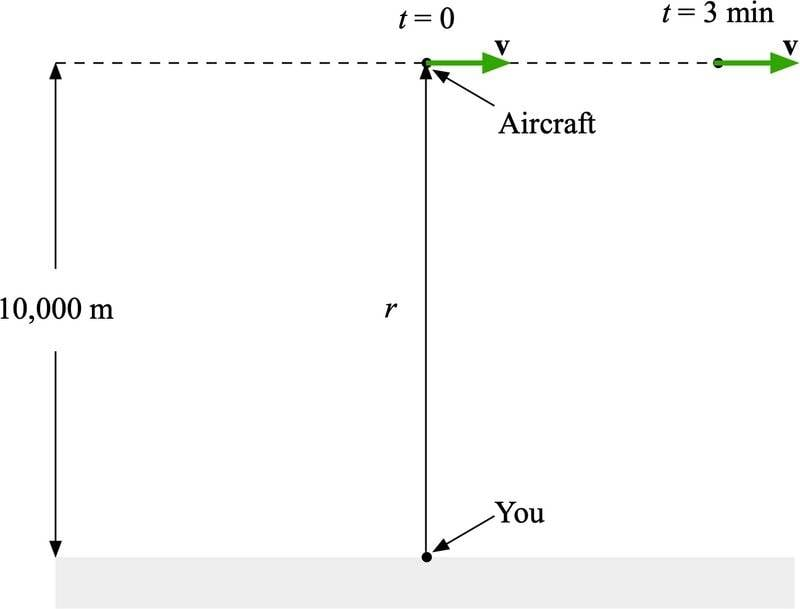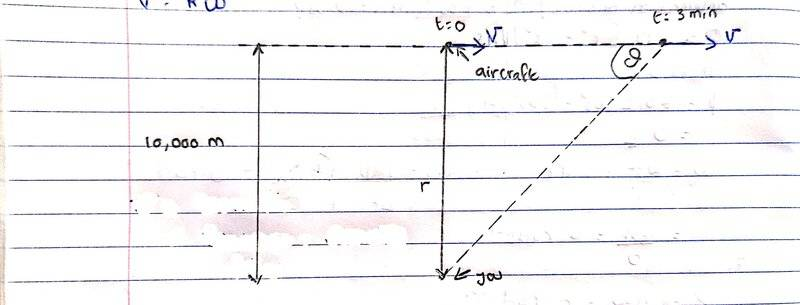# Angular velocity of an airplane

Zoubayr
Homework Statement:
An aircraft passes directly over you with a speed of 900 km/h at an altitude of 10,000 m. What is the angular velocity of the aircraft (relative to you) when directly overhead? Three minutes later?
Relevant Equations:: Angular velocity
Relevant Equations:
Angular velocity
Speed = 900km/hour
tan(α)=900t/10000 α=arctan(900t/10000)
Derivative is 900/(10000+81 t^2)

Staff Emeritus
Homework Helper
Relevant Equations:: Angular velocity
That's not an equation. Equations have equal signs in them.

Speed = 900km/hour
tan(α)=900t/10000 α=arctan(900t/10000)
Derivative is 900/(10000+81 t^2)

Homework Helper
Gold Member
2022 Award
Speed = 900km/hour
tan(α)=900t/10000 α=arctan(900t/10000)
Derivative is 900/(10000+81 t^2)
1. You have divided a speed in km/h by a distance in m. What are the units of the result?
2. You don't need to get into trig. You are only concerned with the instantaneous situation as the plane is directly overhead, so you can approximate for small t.
3. Never plug in numbers straight away. Work symbolically as far as you can. It has many advantages, including allowing others to follow what you are doing.
4. There is an easier way, which may become apparent if you resolve issues 2 and 3.

Zoubayr
That's not an equation. Equations have equal signs in them.

The equation for angular velocity is v=rw

Actually this is not my answer. A friend sent me this. I do not know how to start this question.

Staff Emeritus
Homework Helper
The equation for angular velocity is v=rw.
Well, that's a place to start. Can you identify any of the quantities given in the problem statement with the variables in that expression?

Zoubayr
From the eqn v=rw, only the value of v=900km/hr is given the question. Is the altitude considered as radius?

Staff Emeritus
Homework Helper
From the eqn v=rw, only the value of v=900km/hr is given the question. Is the altitude considered as radius?
In that equation, ##r## represents the distance from the point of reference to the moving object. What do you think? Does the altitude fit the bill?

Zoubayr
I think so but I am not sure

Staff Emeritus
Homework Helper
Making a drawing can help.What do you think now?

•erobz and Lnewqban
Zoubayr
Yes the value of the altitude is the radius r. So by using v=rw and making w sof, we get: w=v/r= (900 x 1000)/ (10,000×3600) = 900,000/ 36,000,000 = 0.025 rad/s for t=0s. But what about when t=3s?

Gold Member
Yes the value of the altitude is the radius r. So by using v=rw and making w sof, we get: w=v/r= (900 x 1000)/ (10,000×3600) = 900,000/ 36,000,000 = 0.025 rad/s for t=0s. But what about when t=3s?
Try drawing a triangle where the angle ##\theta## subtends the horizontal distance traveled by the plane in time ##t##. What is the rate of change of that angle w.r.t. time?

Homework Helper
Gold Member
Yes the value of the altitude is the radius r. So by using v=rw and making w sof, we get: w=v/r= (900 x 1000)/ (10,000×3600) = 900,000/ 36,000,000 = 0.025 rad/s for t=0s. But what about when t=3s?
Note that ##\tan\theta = \dfrac{vt}{r}##. What is the derivative of that with respect to time?

Homework Helper
Try drawing a triangle where the angle ##\theta## subtends the horizontal distance traveled by the plane in time ##t##. What is the rate of change of that angle w.r.t. time?
Or, if coordinates are more your thing, try splitting the velocity up into a radial component directly away from the ground station and a tangential component perpendicular to that.

Zoubayr
Try drawing a triangle where the angle ##\theta## subtends the horizontal distance traveled by the plane in time ##t##. What is the rate of change of that angle w.r.t. time?Is the drawing right?

Homework Helper
Gold Member
I would define as ##\theta## the other acute angle. See post #12. This problem is a one-liner if you answer my question in #12.

Gold Member
View attachment 318159
Is the drawing right?
I had the other angle in mind ( as @kuruman suggested). We should probably ask (based on what @jbriggs444 noted) are you supposed to be using calculus to solve the problem?

Homework Helper
I had the other angle in mind ( as @kuruman suggested). We should probably ask (based on what @jbriggs444 noted) are you supposed to be using calculus to solve the problem?
Even without calculus, one can ask "given a small increment of time, ##\Delta t##, how much does the angle change"? What is the ratio, ##\frac{\Delta \theta}{\Delta t}##?

Homework Helper
Gold Member
Without calculus, I would extract ##~\vec {\omega}~## from ##\vec v=\vec {\omega}\times \vec {r}~## by crossing on the left with ##\vec r.##

•erobz and jbriggs444
Staff Emeritus
•